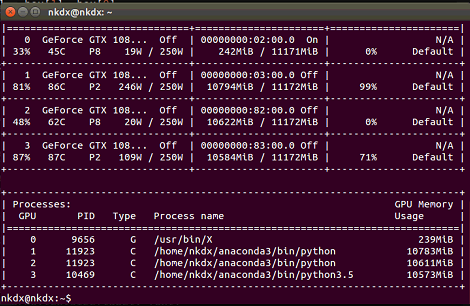# keras（tensorflow）对后端GPU的灵活使用

#### 一、指定对应的GPU（适用于tensorflow，keras）# 设置1秒钟动态刷新一次（1可以调整成别的数字）
watch -n 1 nvidia-smi

import os
#使用第1，2张显卡
os.environ["CUDA_VISIBLE_DEVICES"] = "0, 1"

#### 二、指定GPU用量

##### 1、固定GPU用量
import tensorflow as tf
import keras.backend.tensorflow_backend as KTF

#进行配置，使用30%的GPU
config = tf.ConfigProto()
config.gpu_options.per_process_gpu_memory_fraction = 0.3
session = tf.Session(config=config)

# 设置session
KTF.set_session(session )
##### 2、当然，也可以对GPU用量进行动态调整：
import tensorflow as tf
import keras.backend.tensorflow_backend as KTF

config = tf.ConfigProto()
config.gpu_options.allow_growth=True   #不全部占满显存, 按需分配
session = tf.Session(config=config)

# 设置session
KTF.set_session(sess)

#### 三、keras下的多GPU运行

（1）将模型的输入分为多个子batch
（2）在每个设备上调用各自的模型，对各自的数据集运行
（3）将结果连接为一个大的batch（在CPU上）

• model: Keras模型对象，为了避免OOM错误（内存不足），该模型应在CPU上构建。
• gpus: 大或等于2的整数，要并行的GPU数目。该函数返回Keras模型对象，它看起来跟普通的keras模型一样，但实际上分布在多个GPU上。

import tensorflow as tf
from keras.applications import Xception
from keras.utils import multi_gpu_model
import numpy as np

num_samples = 1000
height = 224
width = 224
num_classes = 1000

# 初始化模型
# (here, we do it on CPU, which is optional).
with tf.device('/cpu:0'):
model = Xception(weights=None,
input_shape=(height, width, 3),
classes=num_classes)

# 在 8 个 GPU上训练模型.
# This assumes that your machine has 8 available GPUs.
parallel_model = multi_gpu_model(model, gpus=8)
parallel_model.compile(loss='categorical_crossentropy',
optimizer='rmsprop')

# Generate dummy data.
x = np.random.random((num_samples, height, width, 3))
y = np.random.random((num_samples, num_classes))

# 这个 fit 操作将会分布在 8 个 GPU上.
# 如果 batch size 为 256, 则每个 GPU 将处理 32 个样本.
parallel_model.fit(x, y, epochs=20, batch_size=256)

'''Trains a simple deep NN on the MNIST dataset.
Gets to 98.40% test accuracy after 20 epochs
(there is *a lot* of margin for parameter tuning).
2 seconds per epoch on a K520 GPU.
'''

from __future__ import print_function

import keras
from keras.datasets import mnist
from keras.models import Sequential
from keras.layers import Dense, Dropout
from keras.optimizers import RMSprop
from keras.utils import multi_gpu_model

batch_size = 128
num_classes = 10
epochs = 20

# the data, shuffled and split between train and test sets
(x_train, y_train), (x_test, y_test) = mnist.load_data()

x_train = x_train.reshape(60000, 784)
x_test = x_test.reshape(10000, 784)
x_train = x_train.astype('float32')
x_test = x_test.astype('float32')
x_train /= 255
x_test /= 255
print(x_train.shape, 'train samples')
print(x_test.shape, 'test samples')

# convert class vectors to binary class matrices
y_train = keras.utils.to_categorical(y_train, num_classes)
y_test = keras.utils.to_categorical(y_test, num_classes)

model = Sequential()

model = multi_gpu_model(model, 2)
model.summary()

model.compile(loss='categorical_crossentropy',
optimizer=RMSprop(),
metrics=['accuracy'])

history = model.fit(x_train, y_train,
batch_size=batch_size,
epochs=epochs,
verbose=1,
validation_data=(x_test, y_test))
score = model.evaluate(x_test, y_test, verbose=0)
print('Test loss:', score)